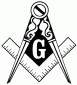# Mechanics of Deformable Bodies: Shear and Moment Equations of Simple Beam with Uniform and Concentrated Loads

3 posts / 0 new
MechanicsMechanics of Deformable Bodies: Shear and Moment Equations of Simple Beam with Uniform and Concentrated LoadsJhun VertThe first thing you need to do is solve for the reactions RB and RD. To find the shear and bending moment equations for each segment, cut a segment at distance x from the left end and write the equation. There are many solved problems in this site that will guide on how to do it step by step. Example of such page is this one: http://www.mathalino.com/node/330. Explore the other pages for more solved problems.

MechanicsThanks sir.

• Mathematics inside the configured delimiters is rendered by MathJax. The default math delimiters are $$...$$ and $...$ for displayed mathematics, and $...$ and $...$ for in-line mathematics.